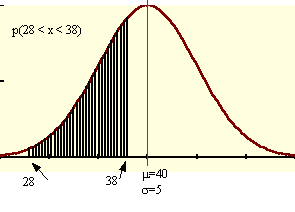### CFA Practice Question

There are 985 practice questions for this topic.

### CFA Practice Question

For x, a random variable from a normal distribution with mean 40 and standard deviation 5, P(28 < x < 38) = ______ (to nearest 0.1%).

A. 35.3%
B. 34.5%
C. 33.6%

The z-score for 38 is (38 - 40)/5 = -0.4. The table value for -0.4, P(x < 38), is 0.3446. The z-score for 28 is -1.2 and the table value is 0.0082.
P(28 < x < 38) = 0.3446 - 0.0082 = 0.3364 or 33.6% (to the nearest 0.1%).User Comment
woojacky z table?
ENDER the z-table for 28 is -2.4 (28-40)/5=-2.4
doze Do I trully need to memorize all the Z-table....? Miserable brazilian life.
BonnieG yeah, z-score is -2.4? this is wrong! also, how can we possibly memorize a z-table, that should be provided.
tenny45 the cfa website (faq) says that we'd need to "estimate" it based on whatever is given (maybe a portion of the table would be given?). But No complete tables will be provided.
kaliokale if CFA wants us to "estimate"... the answers sholdn't be that close to each other.
bobert Yeah, without the table this is impossible.
epizi if it was so easy to memorize this table they wouldn't had provided it in the curriculum notes, at the Appendix B exactly
Becker So P(x>28) is -1.2 or 2.4 ? Why ?
StanleyMo strange,i always get -2.4..
Murrayman You should get -2.4. Also, the z score mentioned above, 0.0082, is the z-score for -2.4, not -1.2.
freda Why not A? ie why not 0.3446+0.0082?
anastasiya I agree with Murrayman.

freda, here is the explanation you need: P(-2.4<z<-0.4)=P(z<-0.4) - P(z<-2.4)= 0.3446 - 0.0082=0.3364
bundy You are looking for the area between 28 and 38 The corresponding Z score for 28 is -2.4 and prob that observation lies below that is .0082. The corresponding Z score for 38 is -.4 and the prob that observation lies below that is .3446. SO the area between the two is the prob of the observation falling btw them hence .3446 - .0082 = 33.6%
bkballa you guys worry too much. There's no way you would be asked to do this without a table. Also keep in mind that the whole quant section has about 24 ques so there might only be 1 or 2 on this particular topic.
johntan1979 Agree with bkballa.
PSVC Z- table extracts are provided for questions to which they will be applied.
ashish100 WE ARE SCREWED!!!!!

Everybody panic so I can score above the 60th percentile. :D jk